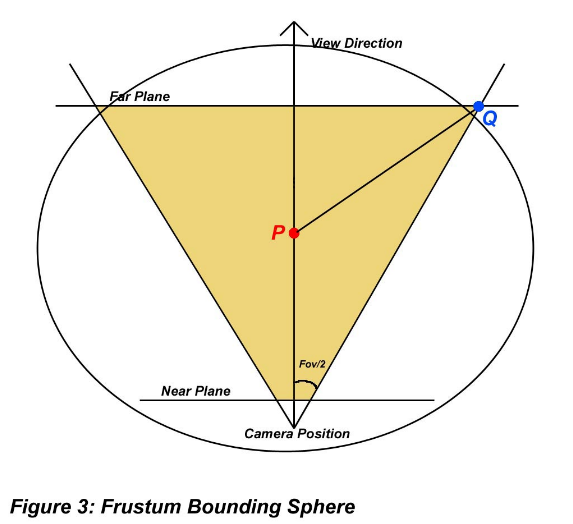# [3D图形学]视锥剔除入门(翻译)### 基本方法1

http://www2.ravensoft.com/users/ggribb/plane%20extraction.pdf

(译注:由于C和N同维数,所以C与N可以直接点乘.我刚开始看到这个公式时很晕乎,后来百度出一篇高中教案,其中写道"利用法向量求点到平面的距离...把点A到平面的距离看成点A与平面内任意一点B所构成的向量在法向量方向上的投影的长度...",这便是C·N的出处,由于坐标系原点不一定在我们的裁面上,所以最后还要+D来修正一下.顺便一提那教案结尾还加了一句"此方法无需技巧,人人都会",我去年买了个表.)

```///判断某球体是否在平截头体内
int Frustrum::ContainsSphere(const Sphere& refSphere) const
{
//球体中心到某裁面的距离
float fDistance;
//遍历所有裁面并计算
for(int i = 0; i < 6; ++i)
{
//计算距离
fDistance = m_plane[i].Normal().dotProduct(refSphere.Center())+m_plane[i].Distance();
//如果距离小于负的球体半径,那么就是外离
return(OUT);
//如果距离的绝对值小于球体半径,那么就是相交
return(INTERSECT);
}
//否则,就是内含
return(IN);
}```

```///判断AABB盒是否在平截头体内
int Frustrum::ContainsAaBox(const AaBox& refBox) const
{
Vector3f vCorner;
int iTotalIn = 0;
//获得所有顶点
refBox.GetVertices(vCorner);
//测试6个面的8个顶点
//如果所有点都在一个的背后,那就是外离
//如果所有点都在每一个面的正面,就是内含
for(int p = 0; p < 6; ++p) {
int iInCount = 8;
int iPtIn = 1;
for(int i = 0; i < 8; ++i) {
//测试这个点
if(m_plane[p].SideOfPlane(vCorner[i]) == BEHIND)
{
iPtIn = 0;
--iInCount;
}
}
//所有点都在p面背后吗?
if(iInCount == 0)
return(OUT); //外离
iTotalIn += iPtIn;
}
//如果iTotalIn是6,那么就都是在正面
if(iTotalIn == 6)
return(IN); //内含
return(INTERSECT); //相交
}```

### 优化1

```///对节点的递归处理
{
//在裁剪前是否需要检测?
if(bTestChildren) {
//首先检测是否是在检测盒内
if(pNode->m_bbox.ContainsPoint(pPovCamera->Position()) == NOT_INSIDE)
{
//先进行球体检测
switch(pPovCamera->Frustrum().ContainsSphere(pNode->m_sphere)) {
case OUT:
return;
case IN:
bTestChildren = false;
break;
case INTERSECT:
//检测立方体是否在视角内
switch(pPovCamera->Frustrum().ContainsAaBox(pNode->m_bbox)) {
case IN:
bTestChildren = false;
break;
case OUT:
return;
}
break;
}
}
}
//[在这里填入判断代码]
}```

### 基本方法2

```///测试两个球体是否相交
bool Sphere::Intersects(const Sphere& refSphere) const
{
//获得一个从目标球心指向本球心的向量
Vector3f vSepAxis = this->Center() - refSphere.Center();
//计算半径和
//简单地说,如果向量的模长小于半径的话,就是相交
//但直接求向量模长的话,需要一次开根操作
//而乘法(平方)操作远比开跟操作要快
//所以我使用摸的平方与半径和的平方作对比
return(true);
//否则就是不相交
return(false);
}```

http://wenku.baidu.com/view/6388b482e53a580216fcfe9c

### 优化2

Yep,你猜到了.那两个算法是用来进一步优化我们的视锥剔除算法的.正如我在上一章的开头所说,平截头体检测其实是很慢的,能避免的话就不要进行与它相关的操作.你应该也注意到球-球检测和球-锥检测要比平截头体检测要快一些.所以我们可以把它们作为预检测算法,利用它们的高速,提前剔除掉那些根本不可能相交的物体,仅让可能相交的物体进行平截头体检测.接下来我们要做的是为平截头体构建一个球体和圆锥.之后在检测时,我们让物体的渲染判定球和平截头体的球进行检测,通过后再与圆锥进行检测,依然通过后则再与平截头体进行检测.这种算法看上去很麻烦,其实对计算机而言计算起来远比直接检测平截头体要快得多.```//计算平截头体球的半径
//首先计算远裁面与近裁面的距离
float fViewLen = m_fFarPlane - m_fNearPlane;
//计算远裁面的高,这里有个问题,FOV通常是从视角原点开始算的,这里应该用m_fFarPlane而不是fViewLen才对,作者的笔误?
float fHeight = fViewLen * tan(m_fFovRadians * 0.5f);
//在横纵比为1时,宽和高一样,否则还要再计算宽...
float fWidth = fHeight;
//确定P点
Vector3f P(0.0f, 0.0f, m_fNearPlane + fViewLen * 0.5f);
//确定Q点
Vector3f Q(fWidth, fHeight, fViewLen);
//获得一个半径向量
Vector3f vDiff(P - Q);
//于是球体半径就是模长了
//然后我们要确定这个球在全局坐标系中的位置
Vector3f vLookVector;
m_mxView.LookVector(&vLookVector);
//计算球体中心在全局坐标中的位置
m_frusSphere.Center() = m_vCameraPosition + (vLookVector * (fViewLen * 0.5f) + m_fNearPlane);```

```// vLookVector是镜头的方向向量
// Position()为获取镜头的位置
// fWidth为屏幕宽度的一半(单位:像素).
// fHeight为屏幕高度的一半(单位:像素).

// 计算FOV三角形的临边
float fDepth  = fHeight / tan(m_fFovRadians * 0.5f);
// 计算从屏幕中点到边角的距离
float fCorner = sqrt(fWidth * fWidth + fHeight * fHeight);
// 计算新的FOV
float fFov = atan(fCorner / fDepth);
// 初始化圆锥
m_frusCone.Axis() = vLookVector;
m_frusCone.Vertex() = Position();
m_frusCone.SetConeAngle(fFov);```

```///对节点的递归处理
//在裁剪前是否需要检测?
if(bTestChildren)
{
//首先检测是否是在检测盒内
if(pNode->m_bbox.ContainsPoint(pPovCamera->Position()) == NOT_INSIDE)
{
//检测我们是否和平截头体的球体相交
if(!pPovCamera->FrustrumSphere().Intersects(pNode->m_sphere))
return;
//检测我们是否和平截头体的圆锥相交
if(!TestConeSphereIntersect(pPovCamera->FrustumCone(), pNode->m_sphere))
return;
//先进行球体检测
switch(pPovCamera->Frustrum().ContainsSphere(pNode->m_sphere))
{
case OUT:
return;
case IN:
bTestChildren = false;
break;
case INTERSECT:
//检测立方体是否在视角内
switch(pPovCamera->Frustrum().ContainsAaBox(pNode->m_bbox))
{
case IN:
bTestChildren = false;
break;
case OUT:
return;
}
break;
}
}
}
//[在这里填入额外的判断和渲染代码]
}```